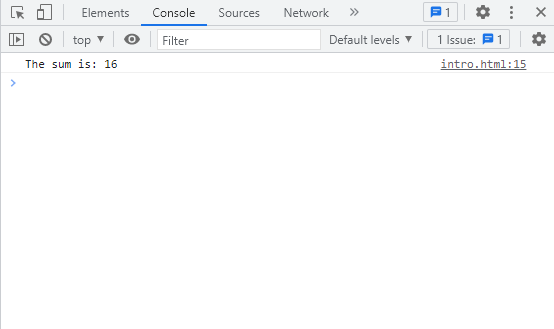in STEMGeeks2 years ago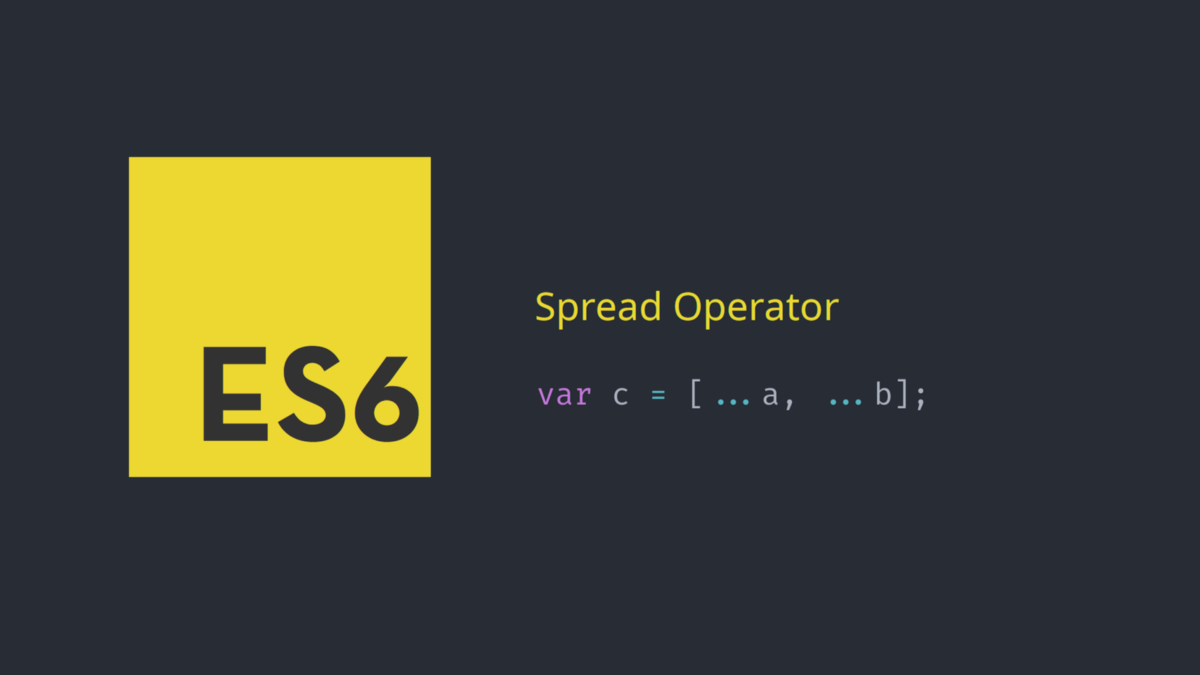Image Source

Spread operator is one of the cool feature introduced in JavaScript ES6 (ECMAScript 6). It is represented by triple dots (...) and is different from the Rest operator in JavaScript although their syntax is similar. Spread operator is used to add element of one array into the another or merge two array into one. In the same way, you can use it to create a copy of an original array so that you can perform operation on it without modifying the original array. And lastly, spread operator helps to pass array as an argument to a function and thus make it easier for array to work with function as well. Lets see an example merging two array into one using the spread operator.

``````<!DOCTYPE html>
<html lang="en">
<script>
var odd_array = [1,3,5,7];
var even_array = [2,4,6,8];
var num_array = [...odd_array, ...even_array];
console.log(num_array);
</script>
<body>
</body>
</html>
``````

If you open console on your browser, you can see the following output: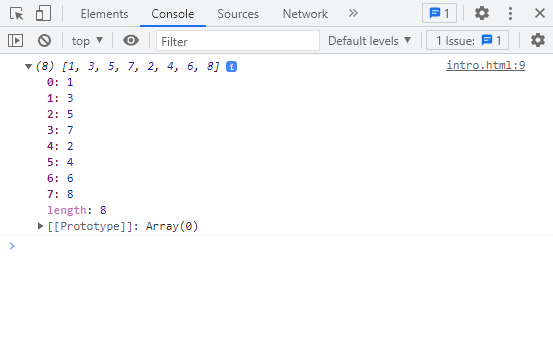Lets see what the output will be if you try to remove that three dots in above code in line number 8. It will be just: `var num_array = [odd_array, even_array];`.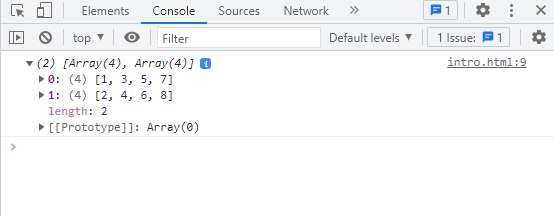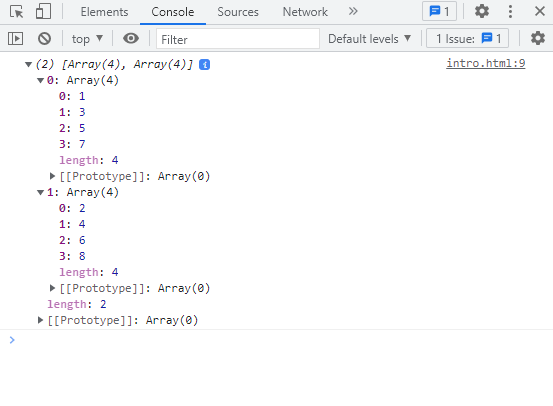You will just be getting an array that consists of another array inside it. So I hope you already know what spread operator does. As the name implies, it spreads the element inside the array. Now lets move ahead on how we can use it as an argument in function.

``````<script>
var num_array = [1,6,5,4];
function findSum(a,b,c,d)
{
console.log("The sum is: " + (a+b+c+d));
}
findSum(...num_array);
</script>
``````

Using spread operator in function argument during function call, it helps to split the individual element in the array and then map it to their respective function parameter. You can see the output as 16 in the console.